# Nonlinear Regression

For an introduction to this topic see the publication by Archontoulis and Miguez (https://doi.org/10.2134/agronj2012.0506) and the book chapter by Miguez, Archontoulis and Dokoohaki ( https://doi.org/10.2134/appliedstatistics.2016.0003.c15).

One of the objectives of those publications was to introduce a large family of nonlinear functions to practicioners in the agricultural research area to a variety of tools that can use to fit data which does not conform to a linear relationship. The feature that distinguishes this approach from others such as ploynomials, splines or gams (to name a few) is that the parameters of the model have biologically meaningful interpretations. In R the approach that makes fitting nonlinear mixed models almost as easy as fitting linear mixed models is the use of self starting functions.

# Index of self starting functions

## Functions in ‘base’ R

stats package

1. SSasymp (Asymptotic)
2. SSasympOff (Asymptotic with an offset)
3. SSasympOrig (Asymptotic through the Origin)
4. SSbiexp (Bi-exponential)
5. SSfol (First order compartment)
6. SSfpl (Four parameter logistic)
7. SSgompertz (Gompertz)
8. SSlogis (Logistic)
9. SSmicmen (Michaelis-Menten)
10. SSweibull (Weibull)

## Functions in this package (nlraa)

1. SSbgf (Beta-Growth Function)
2. SSbgf4 (Four Parameter Beta-Growth Function)
3. SSbgrp (Beta-Growth Reparameterized)
4. SSbg4rp (Four Parameter Beta-Growth Reparameterized)
5. SSdlf (Declining Logistic Function)
6. SSricker (Ricker population growth)
7. SSprofd (Profile of protein distribution)
8. SSnrh (Non-rectangular hyperbola)
9. SSlinp (linear-plateau)
10. SSplin (plateau-linear)
15. SSblin (bilinear)
16. SSexpf (exponential function)
17. SSexpfp (exponential-plateau)
18. SSpexpf (plateau-exponential)
19. SSbell (bell-shaped function)
20. SSratio (rational function)
21. SSlogis5 (five-parameter logistic)
22. SStrlin (tri-linear function)
23. SSexplin (expolinear)
24. SShill-123 (Hill function with 1, 2, or 3 parameters)
25. SSbeta5 (beta temperature response function with 5 parameters)
26. SStemp3 (temperature response function with 3 parameters)

For the reference on the Beta growth function see Yin et al. 2003 (https://doi.org/10.1093/aob/mcg029) and Erratum: (https://doi.org/10.1093/aob/mcg091). For a reference on the beta temperature response function see Yin et al. 1995 (https://doi.org/10.1016/0168-1923(95)02236-Q). For a reference on a use of the declining logistic see Oddi et al. (2019) (and vignette in this package). For the Ricker model see: (https://en.wikipedia.org/wiki/Ricker_model). For the protein profile in the canopy see Johnson et al. (2010) (https://doi.org/10.1093/aob/mcq183).

The SSbgrp function is a reparameterized version of SSbgf. The original SSbgf does not enforce the constraint that t.m < t.e and this makes it somewhat numerically unstable for routine use (but more testing is needed). To recover the original parameters from SSbgrp, take the exponential of lt.e, so t.e = exp(lt.e) and likewise t.m = exp(lt.e) - exp(ldt). There is a similar function for the four parameter Beta growth SSbg4rp.

The main benefit of having these self starting functions is that they can be incorporated in the workflow of package ‘nlme’ which allows for fitting nonlinear mixed models. Although there are other tools available, package nlme is still appropriate for most applications in agricultural, environmental and biological sciences.

### List of available SS (self-start) functions.

apropos("^SS")
##   "SS"          "SSD"         "SSasymp"     "SSasympOff"  "SSasympOrig"
##   "SSbell"      "SSbeta5"     "SSbg4rp"     "SSbgf"       "SSbgf4"
##  "SSbgrp"      "SSbiexp"     "SSblin"      "SSdlf"       "SSexpf"
##  "SSexpfp"     "SSexplin"    "SSfol"       "SSfpl"       "SSgompertz"
##  "SShill1"     "SShill2"     "SShill3"     "SSlinp"      "SSlogis"
##  "SSlogis5"    "SSmicmen"    "SSnrh"       "SSpexpf"     "SSplin"
##  "SSratio"     "SSricker"    "SSsharp"     "SStemp3"     "SStrlin"
##  "SSweibull"

## Prediction, Simulation and Bootstrap

Functions for prediction:

1. predict_nls
2. predict_nlme

Some particularly useful functions which simplify generating simulations:

1. simulate_lm
2. simulate_nls
3. simulate_lme
4. simulate_nlme

Other functions which can perform bootsrapping:

1. boot_lm
2. boot_nls
3. boot_lme
4. boot_nlme

# Datasets

These are the available datasets distributed with this package:

1. “sm”
2. “lfmc”
3. “swpg”
4. “barley”
5. “maizeleafext”
## Sorghum and Maize dataset
data(sm)
ggplot(data = sm, aes(x = DOY, y = Yield, color = Crop)) +
geom_point() +
facet_wrap(~ Input)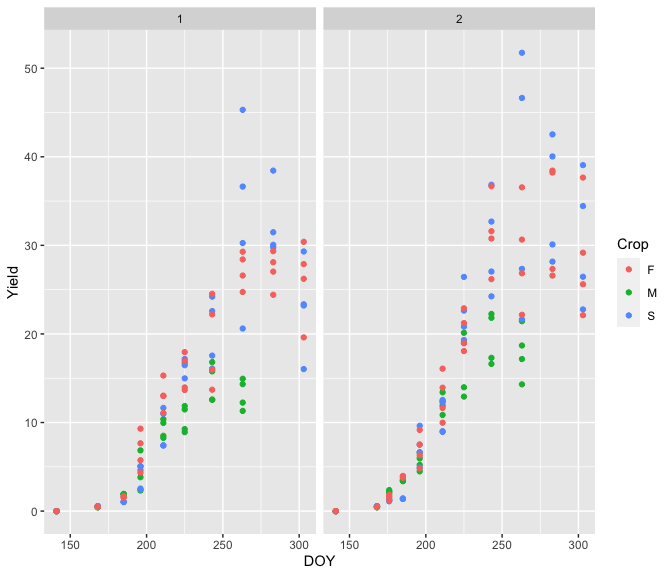## Live fuel moisture content
data(lfmc)
ggplot(data = lfmc, aes(x = time, y = lfmc, color = leaf.type)) +
geom_point() +
ylab("Live fuel moisture content (%)")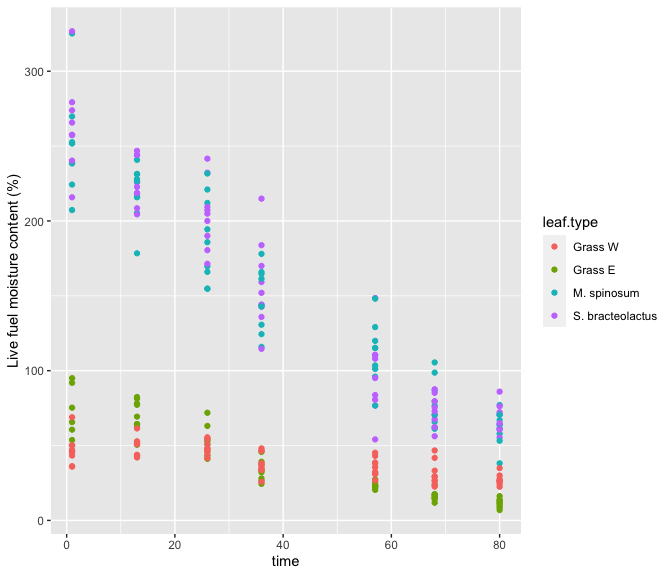## Soil water and plant growth
data(swpg)
ggplot(data = swpg, aes(x = ftsw, y = lfgr)) +
geom_point() +
xlab("Fraction Transpirable Soil Water") +
ylab("Relative Leaf Growth")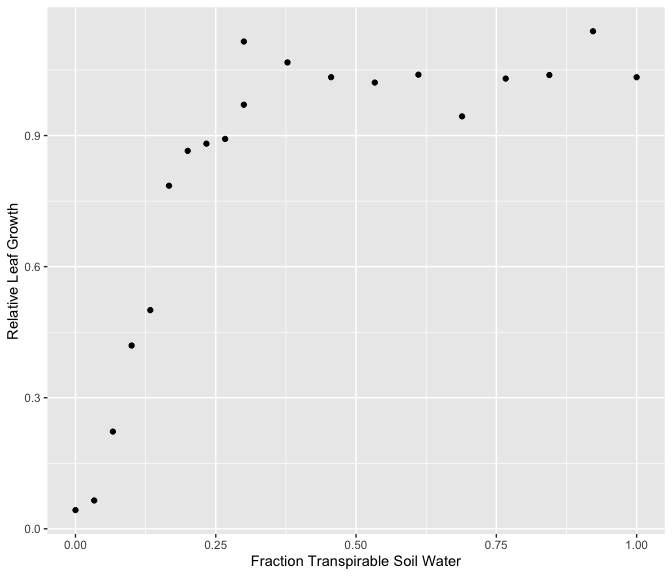## Response of barley to nitrogen fertilizer
## There is a barley dataset also in package 'lattice'
data(barley, package = "nlraa")
ggplot(data = barley, aes(x = NF, y = yield, color = as.factor(year))) +
geom_point() +
xlab("Nitrogen fertilizer (g/m^2)") +
ylab("Grain (g/m^2)")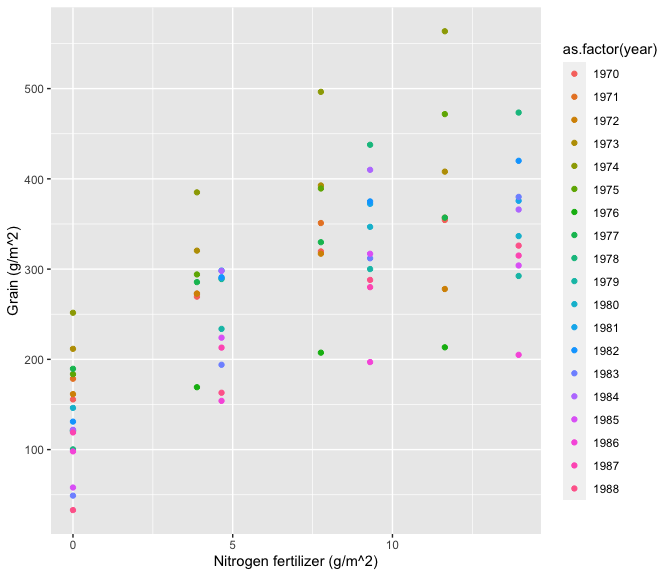## Response of barley to nitrogen fertilizer
## There is a barley dataset also in package 'lattice'
data(maizeleafext, package = "nlraa")
ggplot(data = maizeleafext, aes(x = temp, y = rate)) +
geom_point() + geom_line() +
xlab("Temperature (C)") +
ylab("Leaf Extension Rate (relative)")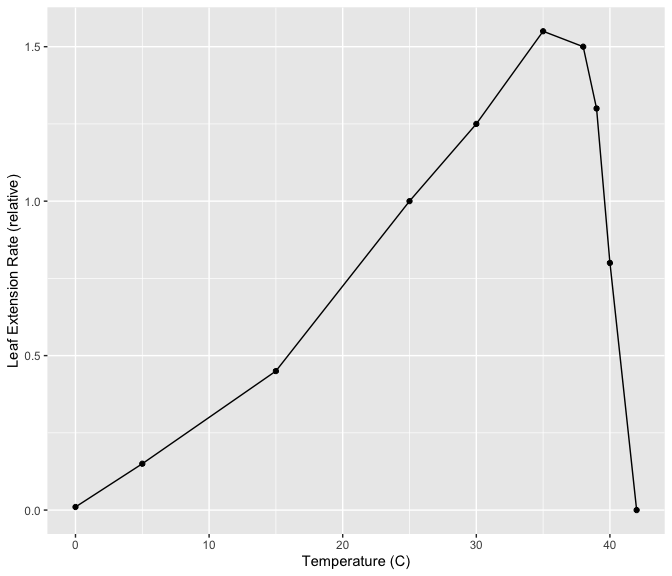# What to do when convergence fails?

The most common issue with nonlinear regression models is related to convergence problems. Convergence problems in nonlinear models can be caused by many different reasons. These are a few of them:

1. The model is not appropriate for the observed data (or viceversa)
2. The model is conceptually correct but there is an error in the formula
3. The model is too complex; a simpler model should be used
4. The model is too simple; a more complex model should be used
5. Starting values are too far from the solution

Model specification (choosing the correct model) is clearly very important when using nonlinear models, the references above and this package are a resource that tries to alleviate this issue.

For convergence problems related to poor starting values there are some alternatives:

1. Try algorithm ‘port’ in function ‘nls’
2. Use an alternative algorithm ‘Levenberg-Marquardt’ in package ‘minpack.lm’ through the function ‘nlsLM’, which can be more robust
3. Use function ‘nls2’ in package ‘nls2’ which uses a ‘brute-force’ approach of searching over a grid
4. Define a custom optimization and use function ‘optim’ in ‘stats’ package (also ‘nlm’ or ‘nlminb’). This option extends the possibility of available algorithms.
5. Manually construct a profile of residual sum of squares as a function of the parameters to understand the relationship which might lead to a better choice for starting values.

## Some common error messages

When fitting nonlinear models some error messages will be commonly encountered. For example,

## Error in nls(y ~ SSratio(x, a, b, c, d), data = dat) :
##  step factor 0.000488281 reduced below 'minFactor' of 0.000976562

Although one option is to reduce minFactor under control in nls it is better to first check that the model is appropriate for the data and that starting values are reasonable. Another option is to use nlsLM from the minpack.lm package, which can be more robust.

Another possible error message is:

## Error in qr.default(.swts * gr) :
##  NA/NaN/Inf in foreign function call (arg 1)

This can be caused by the presence of missing data, which your model cannot handle, or by the presence of zeros in the data that can generate NA/NaN/Inf inside other functions. The solution is to remove missing data and/or zeros.

## Barley example

For background see: (http://miguezlab.agron.iastate.edu/OldWebsite/Research/Talks/ASA_Miguez.pdf).

library(nlme)
data(barley, package = "nlraa")
barley$yearf <- as.factor(barley$year)
barleyG <- groupedData(yield ~ NF | yearf, data = barley)

First step is to create the grouped data object. Then fit the asymptotic regression to each year and the mixed model.

## Fit the nonlinear model for each year
fit.nlis <- nlsList(yield ~ SSasymp(NF, Asym, R0, lrc), data = barleyG)
## Use this to fit a nonlinear mixed model
fit.nlme <- nlme(fit.nlis)
## Investigate residuals
plot(fit.nlme)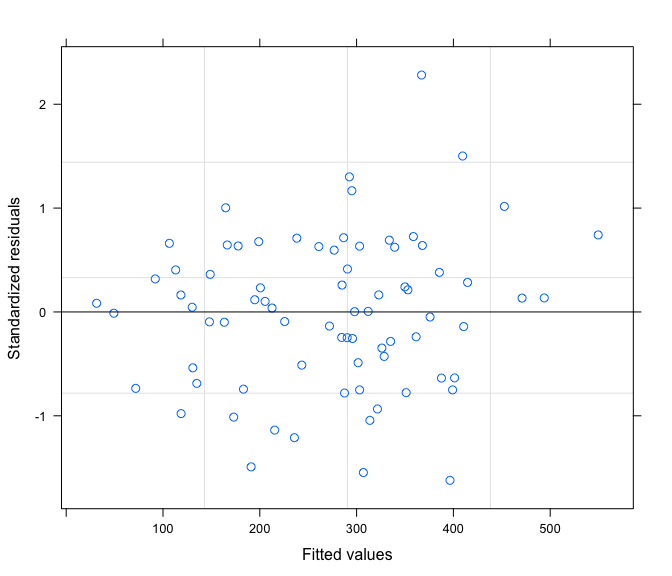## Look at predictions
plot(augPred(fit.nlme, level = 0:1))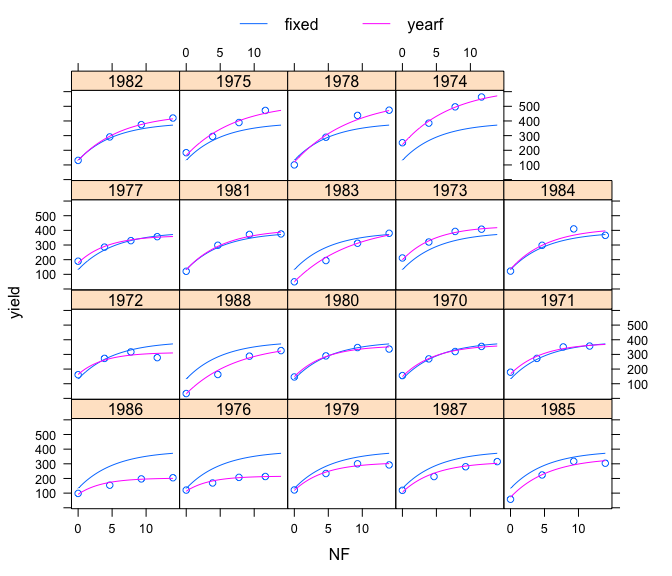## Compute confidence intervals
intervals(fit.nlme)
## Approximate 95% confidence intervals
##
##  Fixed effects:
##           lower      est.      upper
## Asym 337.331378 390.15370 442.976023
## R0   106.772016 131.75233 156.732636
## lrc   -1.890842  -1.66514  -1.439438
## attr(,"label")
##  "Fixed effects:"
##
##  Random Effects:
##   Level: yearf
##                    lower        est.       upper
## sd(Asym)      69.3531468 106.6362143 163.9620225
## sd(R0)        35.5041417  51.0293371  73.3433655
## sd(lrc)        0.1236613   0.3169539   0.8123783
## cor(Asym,R0)  -0.1758864   0.3541783   0.7250264
## cor(Asym,lrc) -0.9574850  -0.7989574  -0.2698169
## cor(R0,lrc)   -0.5375575   0.2143155   0.7763412
##
##  Within-group standard error:
##    lower     est.    upper
## 13.81175 18.79545 25.57744
## A simpler model is possible...

## Other modeling approaches

Other modeling approaches do not use such a rigid specification as the models classically used in nonlinear relationships. Some examples are:

1. splines (packages ‘splines’ or ‘splines2’)
2. gams (package ‘mgcv’)
3. loess (package ‘stats’)
4. quantile regression (packages ’quantreg*’)
5. polynomials (poly in package ‘stats’)
6. segmented regression (segmented in package ‘segmented’)

Although these previous methods are much more flexible than classical nonlinear regression, the traditional approaches have the benefit of being simple and providing parameters with a straight-forward interpretation.

# Contributed packages

I have not tested any of these packages. They are here for reference.

## FlexParamCurve package

See Oswald, Nisbet, Chiaradia and Arnold, MEE, (2012) ( https://doi.org/10.1111/j.2041-210X.2012.00231.x).

1. SSposnegRichards (Positive-Negative Richards)

## sicegar package

Available from CRAN. See: See Umut Caglar, Teufel and Wilke (https://peerj.com/articles/4251/)

Package available at https://github.com/wilkelab/sicegar

## drc package

Dose-response curves package

Ritz C, Baty F, Streibig JC, Gerhard D (2015) Dose-Response Analysis Using R. PLoS ONE 10(12): e0146021. .

This package actually has very useful functions, datasets and examples. In particular drm is an alternative to nls.

## easynls pacakge

Might make it easier to fit and plot a few different models.

## chngpt pacakge

chngpt: threshold regression model estimation and inference. .

## mcp package

mcp: package for multiple change points. Similar to the previous one, but it seems to use a Bayesian approach through JAGS. (https://lindeloev.github.io/mcp/)

## nlsr package

This is an effort to replace ‘nls’ with a better algorithm, but it seems to be still in development. Main function is nlxb.

## propagate package

This provides uncertainty for ‘nls’ objects via function ‘predictNLS’.

## car package

“Companion to Applied Regression”. It has function ‘Boot’ which can do bootstrapping for ‘nls’ objects.

## HydroMe package

Note: As of 2020-12-8 it has been removed from CRAN. This package has functions for fitting water retention curves. It uses the ‘minpack.lm’ package which has a different implementation of the minimization algorithm (Levenberg-Marquardt).

This package has the following selfStart functions:

1. SSfredlund
2. SSgampt
3. SSgard
4. SSgardner
5. SShorton
6. SSkosugi
7. SSomuto
8. SSphilip
9. SSvgm

1. Tani
2. Brook
3. Campbel
4. Expo

## Nonlinear mixed models packages

saemix, nlmixr and brms (Bayesian). I’m planning to review these pacakges in a future version of nlraa.

## Additional material on growth models

1. A unified approach to the Richards-model family for use in growth analyses: Why we need only two model forms. . Might incorporate this function in the package in the future.

2. The use of Gompertz models in growth analyses, and new Gompertz-model approach: An addition to the Unified-Richards family. (At the moment the DOI does not work.)

3. Choosing the right sigmoid growth function using the unified-models approach. .

4. I find this post by Ben Bolker very interesting… https://stats.stackexchange.com/questions/231074/confidence-intervals-on-predictions-for-a-non-linear-mixed-model-nlme